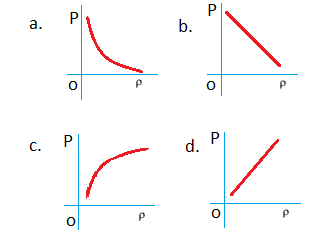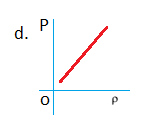# JEE Main Kinetic Theory of Gases Previous Year Questions with Solutions

The Kinetic Theory of Gases was developed by James Clark Maxwell, Rudolph and Claussius to explain the behaviour of gases. It helps in understanding the physical properties of the gases at the molecular level. The Kinetic Theory of Gases is based on the following assumptions.

## Assumptions of Kinetic Theory of Gases

• Gas is a collection of numerous atoms or molecules.
• The space occupied by the molecules of gas in a container is very negligible. Particles are generally far apart such that their inter-particle distance is much larger than the particle size and there is large free unoccupied space in the container.
• Particles are independent. They do not have any (attractive or repulsive) interactions among them.
• The particles are always in constant motion. Because of lack of interactions and the free space available, the particles randomly move in all directions but in a straight line. These molecules always have linear motion.
• Owing to motion, gas particles occupy the total volume of the container whether it is small or big and hence the volume of the container to be treated as the volume of the gases.
• Mean free path is the average distance a particle travels to meet another particle.
• Since the particles are always in motion, they have average kinetic energy proportional to the temperature of the gas
• The free movement of molecules results in collision which is perfectly elastic. Collisions do not change the energy or momentum of the particle.
• Collision of the particles on the walls of the container exerts a force on the walls of the container. Force per unit area is the pressure. The pressure of the gas is thus proportional to the number of particles colliding (frequency of collisions) in unit time per unit area on the wall of the container.

## JEE Main Previous Year Solved Questions on Kinetic Theory of Gases

Q1: A gas mixture consists of 3 moles of oxygen and 5 moles of argon at temperature T. Considering only translational and rotational modes, the total internal energy of the system is

(a) 20 RT

(b) 12 RT

(c) 4 RT

(d) 15 RT

Solution

U = (f1/2)n1RT + (f2/2)n2RT

U = (5/2)(3RT) + (3/2)(5RT)

U = 15RT

Q2: An ideal gas is enclosed in a cylinder at a pressure of 2 atm and temperature, 300 K. The mean time between two successive collisions is 6 × 10–8 s. If the pressure is doubled and the temperature is increased to 500 K, the mean time between two successive collisions will be close to

(a) 4 × 10–8 s

(b) 3 × 10–6 s

(c) 0.5 × 10–8 s

(d) 2 × 10–7 s

Solution

Root mean square velocity

$V_{rms} = \sqrt{\frac{3RT}{M}} = \sqrt{\frac{3P}{\rho }}$

Here,

R is the universal gas constant

M is the molar mass

P is the pressure due to gas

ρ is the density

Vrms$\sqrt{T}$

Vrms ∝ mean free path/ time between successive collisions

And mean free path $Y = \frac{kT}{\sqrt{2\pi \sigma ^{2}P}}$

Vrms ∝ Y/b

Vrms$T/\sqrt{p}\times t$———–(1)

But Vrms$\sqrt{T}$————–(2)

$\sqrt{T}$$T/\sqrt{p}\times t$

t ∝ $\sqrt{T}/p$ $\frac{t_{2}}{t_{1}}=\sqrt{\frac{T_{2}}{T_{1}}\times \frac{P_{1}}{P_{2}}}$ $\frac{t_{2}}{t_{1}}=\sqrt{\frac{500}{300}\times \frac{P_{1}}{2P_{1}}}=\sqrt{\frac{5}{6}}$ $t_{2}=\sqrt{\frac{5}{6}}t_{1}$

t2 ≈ 4 x 10 -8 s

Answer: (a) 4 x 10 -8 s

Q3: The specific heats, CP and CV of gas of diatomic molecules, A, is given (in units of J mol–1 K–1) by 29 and 22, respectively. Another gas of diatomic molecules, B, has the corresponding values 30 and 21. If they are treated as ideal gases, then

(a) A has a vibrational mode but B has none.

(b) A has one vibrational mode and B has two.

(c) A is rigid but B has a vibrational mode.

(d) Both A and B have a vibrational mode each.

Solution

Here Cp and Cv of A are 29 and 22 and Cp and Cv of B are 30 and 21.

γ = Cp/Cv = 1 + 2/f

For A, Cp/Cv = 1 + 2/f ⇒ f=6

Molecules A has 3 translational, 2 rotational and 1 vibrational degree of freedom

For B, Cp/Cv = 1 + 2/f ⇒ f=5

i.e., B has 3 translational and 2 rotational degrees of freedom.

Answer: (a) A has a vibrational mode but B has none.

Q4: Two moles of helium gas is mixed with three moles of hydrogen molecules (taken to be rigid). What is the molar specific heat of mixture at constant volume? (R = 8.3 J/mol K)

(a) 21.6 J/mol K

(b) 19.7 J/mol K

(c) 15.7 J/mol K

(d) 17.4 J/mol K

Solution

Cv1 of helium = (3/2)R

Cv2 of hydrogen = (5/2)R

Cv of mixture = $\frac{2\times \frac{3}{2}R+3\times \frac{5}{2}R}{(2+3)}$

Cv of mixture = 17.4 J/mol K

Q5: The mass of a hydrogen molecule is 3.32 × 10–27/ kg. If 1023 hydrogen molecules strike, per second, a fixed wall of area 2 cm2 at an angle of 45° to the normal, and rebound elastically with a speed of 103 m s–1, then the pressure on the wall is nearly

(a) 2.35 × 103 N m–2

(b) 4.70 × 103 N m–2

(c) 2.35 × 102 N m–2

(d) 4.70 × 102 N m–2

Solution

As pi = pf

Net force on the wall,

F = dp/dt = 2npfcos450= 2nmvcos450

Here, n is the number of hydrogen molecules striking per second.

Pressure = F/A = (2nmvcos450)/Area

= $\frac{2\times 10^{23}\times 3.32 \times 10^{-27}\times 10^{3}\times (1/\sqrt{2})}{2\times 10^{-4}}$ = 2.35 x 103 N m-2

Answer: (c) 2.35 × 102 N m–2

Q6: In an ideal gas at temperature T, the average force that a molecule applies on the walls of a closed container depends on T as Tq. A good estimate for q is

(a) 2

(b) 1

(c) 4/5

(d) 4/7

Solution

Pressure, P = ⅓(mN/V)V2rms

P = (mN)T/V

If the gas mass and temperature are constant then

P ∝ (Vrms)2 ∝ T

So, force ∝ (Vrms)2 ∝ T

I.e., Value of q = 1

Q7: One kg of a diatomic gas is at a pressure of 8 × 104 N/m2. The density of the gas is 4 kg/m3. What is the energy of the gas due to its thermal motion?

(a) 3 × 104 J

(b) 5 × 104 J

(c) 6 × 104 J

(d) 7 × 104 J

Solution

The thermal energy or internal energy is 5 2 U RT for diatomic gases. (degree of freedom for diatomic gas = 5)

But PV = RT

V = mass/density = 1kg/(4 kg/m3) = (¼)m3

P = 8 x 104 N/m2

U = (5/2) x 8 x 104 x ¼ = 5 x 104 J

Answer: (b) 5 × 104 J

Q8: A gaseous mixture consists of 16 g of helium and 16 g of oxygen. The ratio CP /CV of the mixture is

(a) 1.4

(b) 1.54

(c) 1.59

(d) 1.62

Solution

For 16g of helium, n1 = 16/4 = 4

For 16g of oxygen, n2 = 16/32 = ½

For a mixture of gases

Cv =( n1Cv1 + n2Cv2)/(n1 + n2) where Cv = (f/2)R

Cp =( n1Cp1 + n2Cp2)/(n1 + n2) where Cp = ([f/2] + 1)R

For helium, f = 3 ,n1 = 4

For oxygen, f =5, n2 = ½

$\frac{Cp}{Cv}=\frac{(4\times \frac{5}{2}R)+(\frac{1}{2}\times \frac{7}{2}R)}{(4\times \frac{3}{2}R)+(\frac{1}{2}\times \frac{5}{2}R)}=\frac{47}{29}=1.62$

Q9: Cooking gas containers are kept in a lorry moving with uniform speed. The temperature of the gas molecules inside will

(a) increase

(b) decrease

(c) remain the same

d) decrease for some, while the increase for others.

Solution

It is the relative velocities between molecules that are important. Root mean square velocities are different from lateral translation.

Q10: At what temperature is the root mean square velocity of a hydrogen molecule equal to that of an oxygen molecule at 47°C?

(a) 80 K

(b) –73 K

(c) 3 K

(d) 20 K.

Solution

$v_{rms}=\sqrt{RT/M}$ (Vrms)O2 = (Vrms)H2

$\sqrt{\frac{273+47}{32}}= \sqrt{\frac{T}{2}}$

T = 20 K

Q11:The temperature, at which the root mean square velocity of hydrogen molecules equals their escape velocity from the earth, is closest to

[Boltzmann constant, kB = 1.38 × 10–23 J/K,

Avogadro Number, NA = 6.02 × 1026/ kg,

Radius of Earth: 6.4 × 106 m,

Gravitational acceleration on Earth = 10 m s–2]

(a) 104 K

(b) 800 K

(c) 650 K

(d) 3 × 105 K

Solution

The escape speed of the molecule, $v_{e}=\sqrt{2gR}$

Root mean square velocity, $v_{rms}=\sqrt{\frac{3(k_{B}N)T)}{m}}$

So for ve = vrms ⇒ 2gR = 3(kBN)T/m

T = 2gRm/3kBN = (2 x 10 x 6.4 x 106 x 2 x 10-3)/(3 x1.38 x 10-23 x 6.02 x 1023) = 104 K

Q12: Which of the following shows the correct relationship between the pressure ‘P’ and density of an ideal gas at constant temperature?Solution

Ideal gas equation, PV = nRT

As the temperature is constant.

PV = constant

P(m/ρ) = constant

P ∝ ρ (for given m)Q13: The value closest to the thermal velocity of a Helium atom at room temperature (300 K) in m s–1 is [kB = 1.4 × 10–23 J/K; mHe = 7 × 10–27 kg]

(a) 1.3 × 103

(b) 1.3 × 105

(c) 1.3 × 102

(d) 1.3 × 104

Solution

(3/2)kBT = ½mv2

$v=\sqrt{\frac{3k_{B}T}{m}}=\sqrt{\frac{3\times1.4\times 10^{-23}\times 300}{7\times 10^{-27}}}$

= 1.3 x103 ms-1

Q14: An ideal gas has molecules with 5 degrees of freedom. The ratio of specific heats at constant pressure (CP) and at constant volume (CV) is

(a) 6

(b) 7/2

(c) 5/2

(d) 7/5

Solution

An ideal gas has molecules with 5 degrees of freedom, then

Cv = (5/2)R and Cp = (7/2)R

Cp/Cv = (7/2)R/(5/2)R

Cp/Cv = 7/5

Q15: N moles of a diatomic gas in a cylinder is at a temperature T. Heat is supplied to the cylinder such that the temperature remains constant but n moles of the diatomic gas get converted into monoatomic gas. What is the change in the total kinetic energy of the gas?

(a) 0

(b) (5/2)nRT

(c) (½)nRT

(d) (3/2)nRT

Solution

Initial kinetic energy of the system Ki = (5/2)RTN

Final kinetic energy of the system Kf = (5/2)RT(N-n) + (3/2)RT(2n)

Kf – Ki =ΔK = nRT(3 – 5/2) = (½)nRT